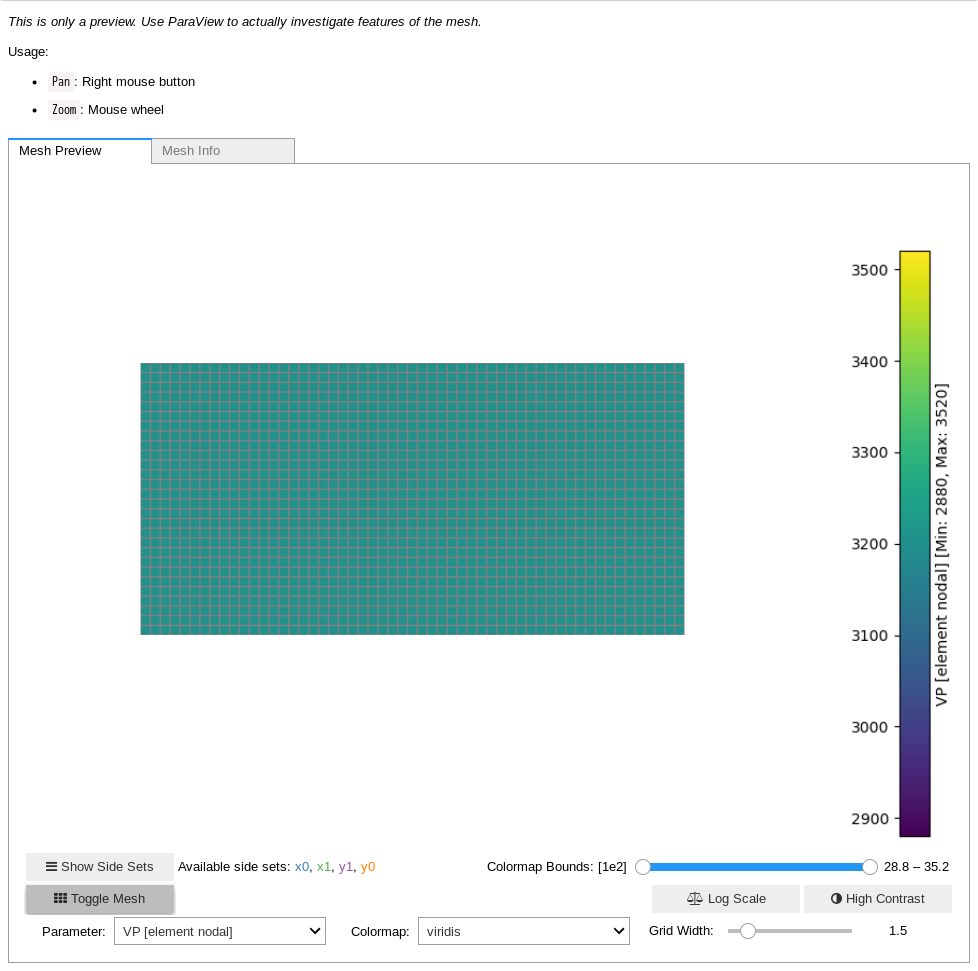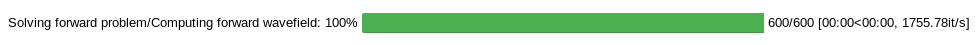Version:

This documentation is not for the latest stable Salvus version.This tutorial is presented as Python code running inside a Jupyter Notebook, the recommended way to use Salvus. To run it yourself you can copy/type each individual cell or directly download the full notebook, including all required files.# Lamb's Problem

Copy
%matplotlib inline

# This line helps with tab-completion of the simple_config objects.
# The IPython/Jupyter project default to a differnet inference based
# tab completion engine which unfortunately does not yet fully work
# with SalvusFlow. This is completely optional and a convenience
# option.
%config Completer.use_jedi = False

## Introduction

This tutorial recreates Lamb's problem without using SalvusProject. SalvusProject was designed to take a more application-driven view and to automatically handles many tasks concerning meshing, bookkeeping, and reproducibility. Under the hood, it calls them same API that we demonstrate in this tutorial. For certain use cases that SalvusProject can not handle yet, this tutorial might be a good starting point to learn about the low-level interface.
An accurate solution to the wave equation is a requirement for a wide variety of seismological research. In this tutorial, we will validate the accuracy of Salvus_Compute_ by comparing numerically calculated seismograms to semi-analytical solutions of Lamb's Problem in 2-D. In addition to giving us confidence in the synthetic data we will use in future tutorials, it also gives us a chance to gently learn some of the key features of the Salvus API.
Lamb's problem is concerned with the behavior of the elastic wave equation in the presence of a half-space bounded by a free-surface condition. In our solution we expect both direct arrivals and those reflected from the free-surface, along with a contribution from the 2-D Rayleigh wave. To validate the solutions generated with Salvus, we will compare our results with semi-analytical ones computed using EX2DDIR. We'll consider a half-space bounded at $y=2000$, and excite waves using a Ricker source with a center frequency of 15 Hz. This setup keeps compute times very low, while also allowing for a fair amount of wavelengths to propagate within our domain. To get started, let's first import all the Python tools we'll need.
# Standard Python packages
import os
import toml
import numpy as np
import matplotlib.pyplot as plt

# Workflow management.
from salvus.flow import api

# Specific objects to aid in setting up simulations.
from pyasdf import ASDFDataSet
from salvus.mesh.simple_mesh import basic_mesh
import salvus.flow.simple_config as sc

SALVUS_FLOW_SITE_NAME = os.environ.get("SITE_NAME", "local")

## Meshing the domain

While future tutorials will see us generating meshes which are a bit more exciting, in this example we will be working with a simple rectangular grid. As a first step we'll import the basic_mesh package from Salvus Mesh. This allows us to quickly generate simple layered and homogeneous meshes in 2- and 3-D. For a detailed overview of the features available in the mesher, please check out the relevant tutorials online. We'll now set some basic parameters which will describe our simulation. As described above, we've preselected some elastic parameters which will suit our purposes well.
# Domain setup (m).
max_x = 2000.0  # Distance in meters.
max_y = 1000.0  # Distance in meters.
max_frequency = 25.0  # Frequency in Hz.

# Material properties.
vp = 3200.0  # P-wave velocity in m/s.
vs = 1847.5  # S-wave velocity in m/s.
rho = 2200.0  # Density in kg / m^3.
With these basic parameters set, we have the minimum required data to generate an elastic mesh. Below we use the CartesianIsotropicElastic2D to auotmatically generate and return our mesh object. Basic in-notebook visualization is available for all UnstructuredMesh objects returned from Salvus Mesh -- an example is given in the cell below. Of course nothing really beats the fully-featured 3-D visualization options offered in a package like Paraview. To visualize Salvus meshes in this and related programs, you can write the mesh out to a file on disk. The function write_h5_tensorized_model will write the mesh data to an HDF5 file of your choice, along with a XDMF file which provides a high-level description of the HDF5 data. It is this XDMF file which should be opened in Paraview.
# Generate a mesh using the "simplified" meshing interface.
mesh = basic_mesh.CartesianHomogeneousIsotropicElastic2D(
vp=vp,
vs=vs,
rho=rho,
x_max=max_x,
y_max=max_y,
max_frequency=max_frequency,
).create_mesh()

# Visualize the mesh in the notebook.
mesh<salvus.mesh.unstructured_mesh.UnstructuredMesh at 0x7fda09245310>
# Write the mesh to a file which can be visualized in Paraview.
# Open the associated lambs_problem_mesh.xdmf to visualize.
mesh.write_h5("lambs_problem_mesh.h5")

## Setting up a simulation

With our mesh generated we can now go ahead and set up our first simulation.

As a first step we need to set up a source and a few receivers. To faciliate this we'll use several of the objects imported from Salvus Flow above, specifically CartesianVectorSource2D and CartesianReceiver2D. The analytic benchmark we will be comparing to used 2-D vector source with components $[0, -1e10]$ and a relative position of $[1000, 500]$. Here, forces are specified in Newton-meters, with positions in meters. We will choose a Ricker wavelet with a center frequency of $14.5$ Hz as a source time function. For receivers, we'll set up a line of 5 equidistantly spaced between $x=800$ m and $x=1200$ m, at a y position of 1010 m. We need to specify a unique station code for each receiver, as well as a list of which fields we'd like the receivers to record (displacement in this case).
# Sources.
fx, fy = 0.0, -1e10  # Source components (Nm)
sx, sy = 1000.0, 500.0  # Source position (m)
stf = sc.source.stf.Ricker(center_frequency=14.5)  # Center frequency in Hz.
source = sc.source.cartesian.VectorPoint2D(
x=sx, y=sy, fx=fx, fy=fy, source_time_function=stf
)

nr = 5  # Number of receivers.
ry0 = 800.0  # Receiver y-value.
rx0 = 1010.0  # x-value of first receiver.
rx1 = 1410.0  # x-value of last receiver.
x=x, y=ry0, station_code=f"{_i:03d}", fields=["displacement"]
)
for _i, x in enumerate(np.linspace(rx0, rx1, nr))
]

### Input file generation

We can generate the input options for Salvus Compute. To do this, we'll use the WaveformSimulation object which we imported above. This object helps to encapsulate the myriad of options which we can pass to the solver. We can get started by initializing a new WaveformSimulation object with the mesh, source, and receivers we've generated above. Since we'll be comparing to a semi-analytic solution which was created on a specific time-axis, we also need to explicitly set the start, end, and time step of the simulation to match what is expected, although note that in general the time-step can be automatically determined from the mesh. Finally, when our simulation object is ready to go, we can call the .validate() method which will do some quick checks to ensure that the input file will be accepted by Salvus Compute.
# Set up a waveform simulation object.

# Modify the start-time, end-time, and time-step of the simulation.
sim.physics.wave_equation.end_time_in_seconds = 0.52
sim.physics.wave_equation.time_step_in_seconds = 1e-3
sim.physics.wave_equation.start_time_in_seconds = -0.08

# sim.output.volume_data.filename = "wavefield.h5"
# sim.output.volume_data.format = "hdf5"
# sim.output.volume_data.fields = ["displacement"]
# sim.output.volume_data.sampling_interval_in_time_steps = 10

# Make sure that Salvus _Compute_ will accept the current options.
sim.validate()

## Running a simulation

Before continuing, ensure that you've completed the necessary Salvus Flow setup as described in the installation instructions.
With everything ready to go, it's now time to run our first simulation! Salvus Flow's api.run command takes a few arguments worth describing:
• site_name: This is an identifier which tells Flow whether you're running on your local machine, some remote cluster, or perhaps the old chess computer in your grandfather's basement. As long as Salvus has been set up correctly on the specified site all data transfers to / from the local or remote machine will happen automatically. Additionally, if a job management system is present on the remote site Flow will monitor the job queue. Here we're using the site name "local". If you gave your site a different name, please update the site_name parameter below.
• input_file: This expects the WaveformSimluation object which we generated above.
• ranks: This is the number of MPI ranks the job will run on, and can range from 1 to whatever your license will allow.
• output_folder: It is here where all the output files of the simulation will be copied.
• get_all: Salvus can produce a lot of output! The get_all flag tells Flow that we want to fetch everthing which the simlulation creates.
All that's left now is to spin up the simulation on a site of your choosing.
api.run(
input_file=sim,
site_name=SALVUS_FLOW_SITE_NAME,
ranks=4,
wall_time_in_seconds=60,
output_folder="output",
get_all=True,
)
SalvusJob job_2307031227924876_cf33aa1af6 running on local with 4 rank(s).
Site information:
* Salvus version: 0.12.12
* Floating point size: 32* Downloaded 51.7 KB of results to output.
* Total run time: 1.03 seconds.
* Pure simulation time: 0.54 seconds.

<salvus.flow.sites.salvus_job.SalvusJob at 0x7fda04dd0f90>

## Matching Lamb's problem

With our first simluation successfully completed, it's now time to take a look at the results. By default Salvus outputs seismograms in the ASDF file format, which can easily be read by Obspy. Below, I've written a simple utility function which takes a Salvus output file, and plots the results against the pre-computed semi-analytical solution.
def plot_asdf_seismograms(asdf_file, axes):
"""
A simple utility function to plot traces side-by side with a
semi-analytic solution to Lamb's problem.
:param asdf_file: ASDF datafile containing receivers.
:param axes: Axes on which to plot.
"""
for i, waveform in enumerate(asdf_file.waveforms):
for j, cmp in enumerate(["X", "Y"]):
trace = waveform.displacement.select(component=cmp)
axes[j, i].plot(trace.data)

for a in axes[:, 0]:
a.set_ylabel("Displacement (m)")
for a in axes[1, :]:
a.set_xlabel("Time sample")
With this function defined, we can now see how well our simulation has done!
fig, axes = plt.subplots(2, 5, sharex="col", sharey="row", figsize=(20, 5))
with ASDFDataSet("data/force_verticale.h5", mode="r") as ex2ddir, ASDFDataSet(
plot_asdf_seismograms(ex2ddir, axes)